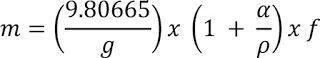# How to Convert Weights to Force Standards

## How to Convert Weights to Force StandardsThe mass of a body relates to the amount of material it contains.

Forces are defined by Newton's second law of motion as expressed in the equation:

F = ma

Since it is impractical to make an accurate determination of force directly from this equation - at least as a routine procedure - it is customary to establish force standards directly from the attraction of the earth on known masses."  - D.R. Tate, a scientist at NIST

The concept of a pound force (lbf) requires the determination of gravity at a fixed location.

### How to Convert Weights to Force Standards - Gravity Determination for a Fixed Location

The actual value of gravity varies over the surface of the Earth from around 983.2 cm/s2 over the poles to 978.0 cm/s2 at the equator.

To find gravity for a fixed location firms or universities can be hired to come to your location to measure gravity.  Typically this will result in a measurement of g accurate to +/- 0.5 mgals.  Alternatively, gravity for your location can be calculated free of charge using online resources.  These values are typically accurate to 5 ppm for anywhere in the US.

### Steps to Find Gravity Using Online Resources:

1. Find your longitude, latitude, and elevation here http://www.geoplaner.com/
2. Calculate your local gravity http://geodesy.noaa.gov/cgi-bin/grav_pdx.prl

Note:  The expanded uncertainty from this calculation is likely to be within 5 ppm anywhere in the US.  This uncertainty value (as a maximum), or the actual reported value, belongs in any uncertainty budget for force, etc. Of course, the mean value of a report must also be applied to the actual measurement data as a correction.

### How to Convert Weights to Force Standards - Weight Adjustment Formula for Weights Used as Force Standards

Because gravity is not a constant over the surface of the earth, weights used as force standards must be adjusted using the gravity value at a fixed location where they are to be used.  They are adjusted so the mass of the weight will produce the required force.The Formula to Determine the Mass Needed to Obtain the Required Force is as Follows:Where:
m =  mass of the weight  (true mass)

g  =  gravity at a fixed location, m/s2.  The force that attracts a body toward the center of the earth, or toward any other physical body having mass.  For most purposes, Newton's laws of gravity apply, with minor modifications to take the general theory of relativity into account.
It is very important for the gravity value for the location where the weight is to be used to be established.  Not using the correct gravity for the location will result in significant errors, up to twenty times the 0.005% allowed by ASTM E74-13a.

a  =  air density - Mass per unit volume of air (kg/m 3)

p  =  material density - A quantitative expression of the amount of mass contained per unit volume. The standard unit is the kilogram per meter cubed (kg/m 3 or kg)

f  =  required force

Note: Air Buoyancy is an upward force exerted by pressure in the air that opposes the weight of an object.

### How to Convert Weights to Force Standards - Calculation Example

1 newton = 101.971621 grams-force
mass = (9.80665/g)(1 + (a/p)f
g = local gravity in m/s2
a = air density   = 0.0012 g/cm 3
p = density of weight material =  7.9 g/cm 3
f = force

9.79957 m/s2 local gravity from http://geodesy.noaa.gov/cgi-bin/grav_pdx.prl
mass =(9.80665/9.79957)(1+(0.0012/7.9)*101.971621  = 102.0607941 grams

Note:  This example calculation was performed using a standard value for air density and material density. To minimize uncertainty, the actual value for the air density at the place of use and the density of the material should be used.

### Converting Force Standards to Mass (Force Transducers)

Converting Force to Mass requires knowing the local gravity where the weighing takes place.

The mass correction factor can be calculated to convert force to mass when the transfer standard is used at a location where the gravity is known as follows:

Mass Correction Factor = (9.80665/g)

where:
g  = gravity at the place of weighing, m/s2  - The gravity value at the place of weighing can be obtained from NOAA as described at the beginning of this article.

To determine Mass when a weighing is performed at the known gravity value  multiply the applied force by the Mass Correction Factor as follows:

Mass = Force x Mass Correction Factor

Note:  This formula works well for force transfer standards.  This formula is for true or apparent mass.   Most calibration service providers provide certificates using conventional mass. Weights require a different formula to determine the mass needed to produce a required force.

### True Mass

The international prototype kilogram, on which the mass scale throughout the world is realized, is defined as a true mass of exactly 1 kilogram. Most high-accuracy comparisons are performed on a true mass basis, but the values are usually converted to conventional mass values when quoted on a certificate.

### Conventional Mass

When weight is calibrated the mass value quoted on its certificate of calibration is normally a conventional mass value - appropriate where the value is determined by weighing the item in air in accordance with International Recommendation OIML R 33. The recommendation says formally: For a weight at 20 °C, the conventional mass is the mass of a reference weight of a density of 8 000 kg/m3 that it balances in the air of density 1.2 kg/m3.

Morehouse has written a full guidance document with detailed information on How to Convert Weights to Force Standards

#How to Convert Weights to Force Standards

• We're committed to your privacy. Morehouse Instrument Company uses the information you provide to us to contact you about our relevant content, products, and services. You may unsubscribe from these communications at any time. For more information, check out our Privacy Policy.